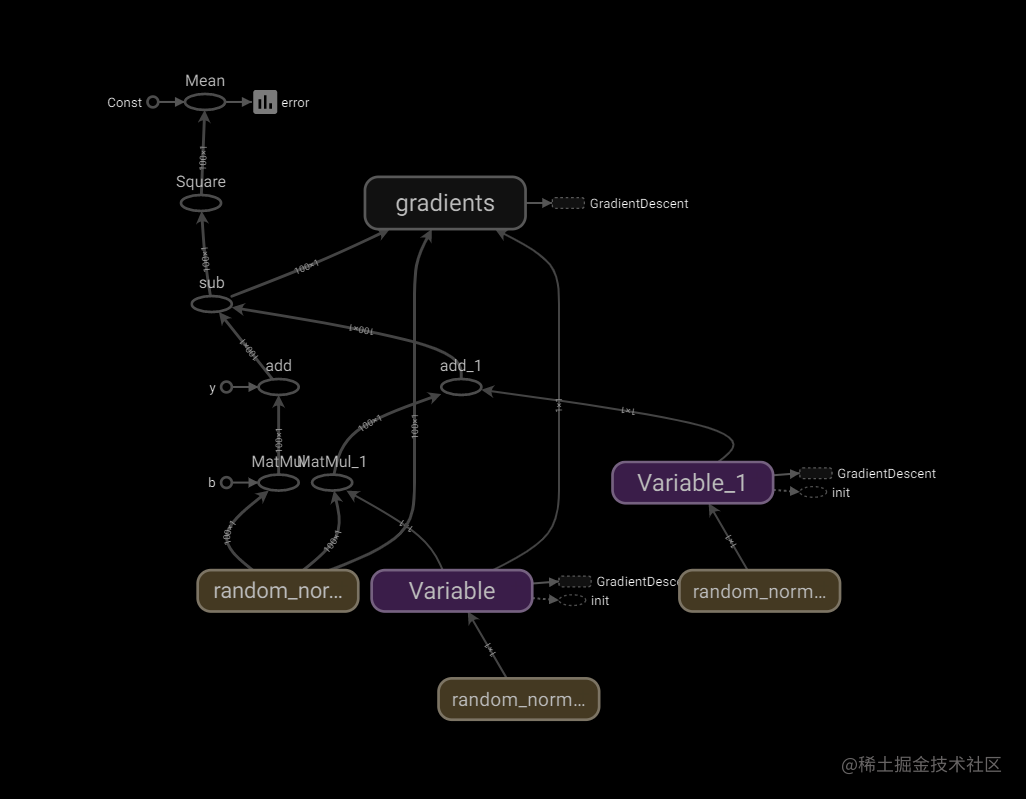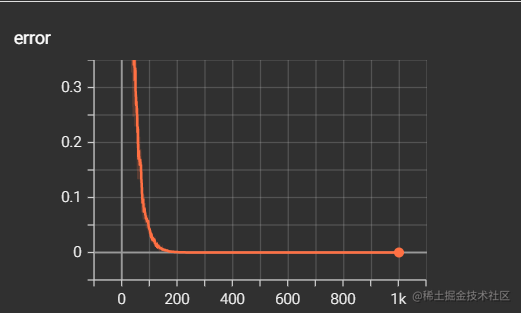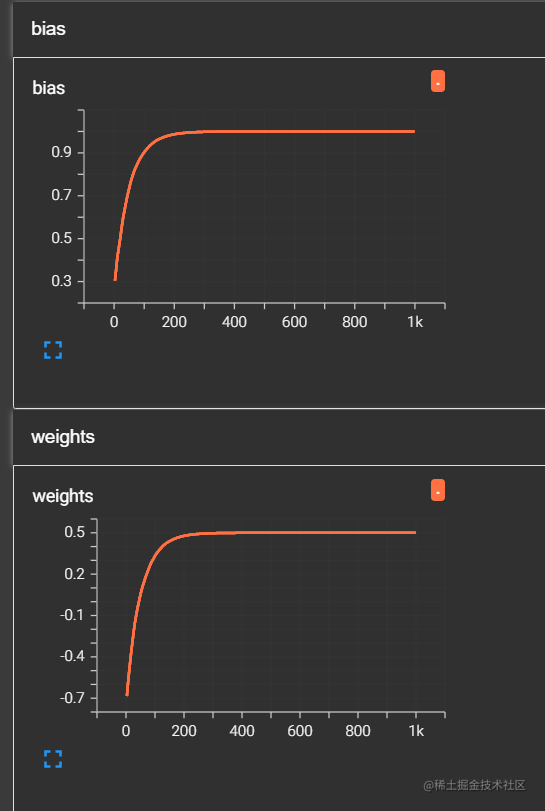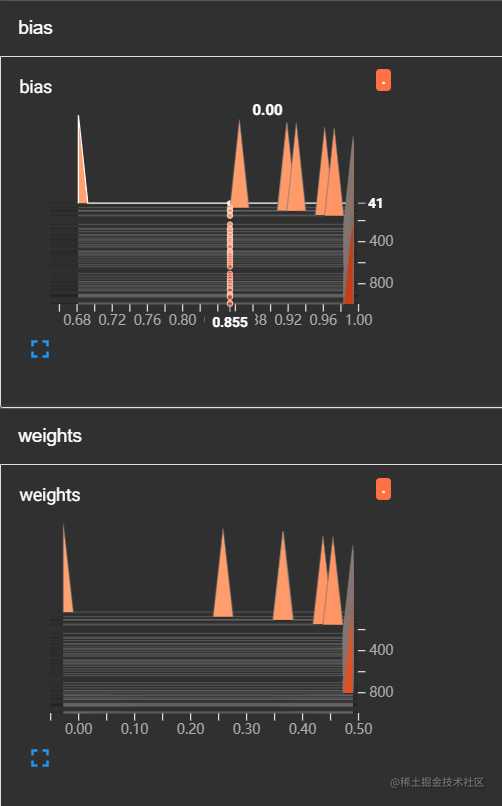# 【深度學習】TensorFlow線性迴歸案例演示（3）

highlight: a11y-dark theme: nico

# 3. 程式碼演示

### 步驟1：匯入所需庫

```ipython import tensorflow as tf import tensorflow.compat.v1 as tf tf.disable_v2_behavior()```

### 步驟2：線性迴歸並收集變數

• 我們在`./File/linear`目錄下建立了事件檔案，用來儲存變數中間結果
• 需要在訓練時的每一次迴圈後將變數寫入事件
• 本案例演示中，主要用到收集變數與變數合併寫入事件，最終得到視覺化結果
• 主要流程為：

1. 建立事件
2. 收集變數
3. 合併變數
4. 寫入變數 ```ipython def linear_regression(): """ 實現線性迴歸 """

# 1.1 準備資料

X = tf.random_normal(shape=[100,1]) y_true = tf.matmul(X, [[0.5]]) + 1 # matmul矩陣運算

# 用變數來定義

weights = tf.Variable(initial_value=tf.random_normal(shape=[1,1])) # 需要給一個初始設定：正態分佈隨機初始值；一行一列 bias = tf.Variable(initial_value=tf.random_normal(shape=[1,1])) y_predict = tf.matmul(X, weights) + bias

# 1.3 損失函式

error = tf.reduce_mean(tf.square(y_predict-y_true))

# 2) 收集變數

tf.summary.scalar("error", error) tf.summary.histogram("weights", weights) tf.summary.histogram("bias", bias)

# 3) 合併變數

merged = tf.summary.merge_all()

# 2. 初始化變數

init = tf.global_variables_initializer()

# 3. 開啟會話

with tf.Session() as sess: sess.run(init)

``````# 1) 建立事件檔案
file_writer = tf.summary.FileWriter("./File/linear", graph=sess.graph)

# 檢視初始化模型引數的值
print("訓練前模型引數為：權重%f, 偏置%f, 損失%f" %(weights.eval(), bias.eval(), error.eval()))

# 4. 開始訓練
for i in range(1000):
sess.run(optimizer)
print("第%d次訓練後模型引數為：權重%f, 偏置%f, 損失%f" %(i+1, weights.eval(), bias.eval(),error.eval()))

# 執行合併變數操作
summary = sess.run(merged)
# 將每次迭代後的變數寫入事件檔案
``````

return None

linear_regression() ``` 經過1000輪訓練得到的結果如下圖所示：### 步驟3：TensorBoard視覺化File檔案中是剛才儲存變數的事件，需要Tensorboard將其開啟檢視。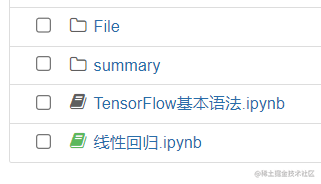`cd 檔案儲存上級目錄path`

`tensorboard --logdir=./File/linear/`